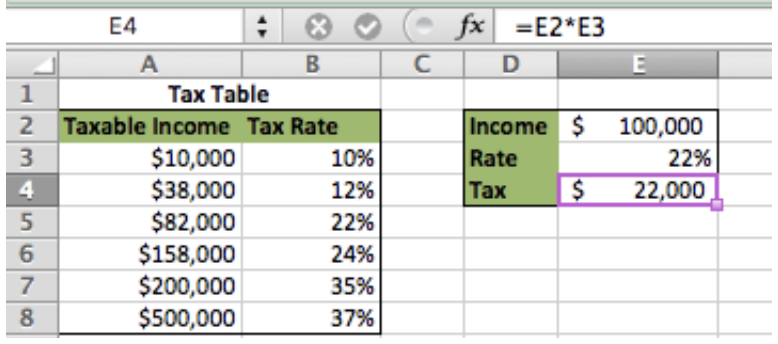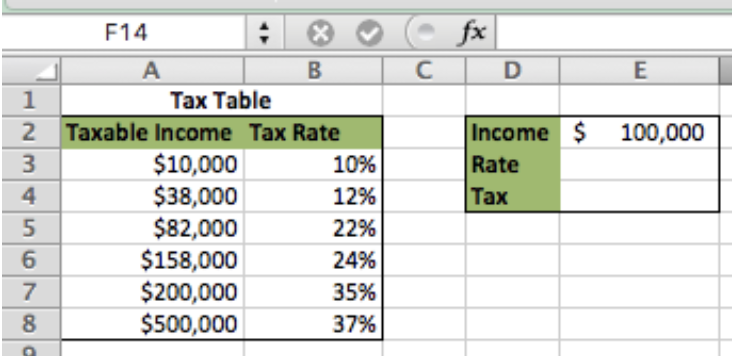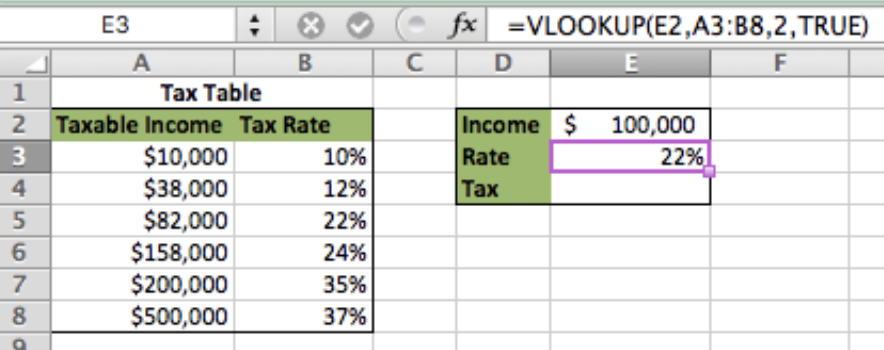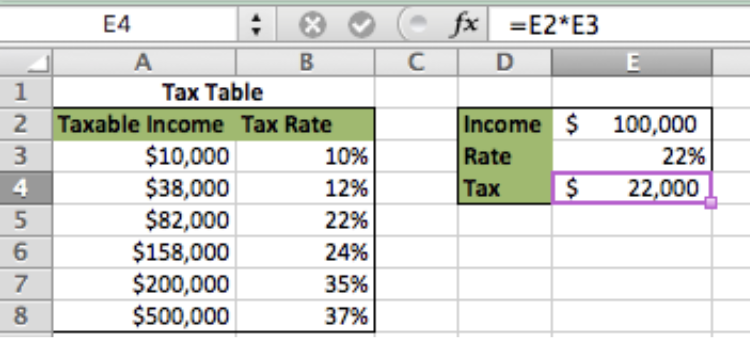Get instant live expert help with Excel or Google Sheets“My Excelchat expert helped me in less than 20 minutes, saving me what would have been 5 hours of work!”

#### Post your problem and you'll get expert help in seconds

Your message must be at least 40 characters
Our professional experts are available now. Your privacy is guaranteed.

# How to Do a Basic Tax Rate Calculation with VLOOKUP

A VLOOKUP formula can be used to calculate a tax rate relative to income. After following a few simple steps, you will be able to quickly calculate Tax based on income levels.Figure 1 – Final Solution

## Setting up the Data

• First, we setup our data so that the taxable income is in Column A and the Tax Rate in column B
• Enter the Income in cell E2 and leave cells E3 and E4 blank for now
• In this example, the Income used for the lookup is \$100,000Figure 2 – Setting up the Data

## Calculate Tax Rate Using VLOOKUP

The VLOOKUP function finds the adjacent value based on the specified lookup value

• The basic formula is `=vlookup(lookup value, table array, column to return, Approximate Match [True/False])`
• In cell E3 type this formula `=VLOOKUP(E2,A3:B8,2,TRUE)`

We can see in this example that the formula returned a Tax Rate of 22% because \$100,000 falls between \$82,000 and \$158,000. If we were to put “False” in the Approximate Match piece of the formula, it would not have calculated properly since \$100,000 doesn’t match any of the values in Column A exactlyFigure 3 – Applying the VLOOKUP formula on Tax Table

*Now, all we have to do is apply the Tax Rate to the Income in order to calculate the Tax.

## Calculate Tax Based on Rate

• The calculation for Tax is Income x Rate = Tax
• In cell E4 type this formula `=E2*E3`Figure 4 – Calculating the Tax

## Instant Connection to an Expert through our Excelchat Service:

Most of the time, the problem you will need to solve will be more complex than a simple application of a formula or function. If you want to save hours of research and frustration, try our live Excelchat service! Our Excel Experts are available 24/7 to answer any Excel question you may have. We guarantee a connection within 30 seconds and a customized solution within 20 minutes.

### Did this post not answer your question? Get a solution from connecting with the expert.Another blog reader asked this question today on Excelchat:## Subscribe to Excelchat.coAnother blog reader asked this question today on Excelchat: